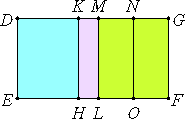# Proposition 61

The square on the first bimedial straight line applied to a rational straight line produces as breadth the second binomial.

Let AB be a first bimedial straight line divided into its medials at C, of which medials AC is the greater. Let a rational straight line DE be set out, and let there be applied to DE the parallelogram DF equal to the square on AB, producing DG as its breadth.

I say that DG is a second binomial straight line.

Make the same construction as before.Then, since AB is a first bimedial divided at C, therefore AC and CB are medial straight lines commensurable in square only, and containing a rational rectangle, so that the squares on AC and CB are also medial.

Therefore DL is medial. And it was applied to the rational straight line DE, therefore MD is rational and incommensurable in length with DE.

Again, since twice the rectangle AC by CB is rational, therefore MF is also rational.

And it is applied to the rational straight line ML, therefore MG is also rational and commensurable in length with ML, that is, DE. Therefore DM is incommensurable in length with MG.

X.36

And they are rational, therefore DM and MG are rational straight lines commensurable in square only. Therefore DG is binomial.

It is next to be proved that it is a second binomial straight line.

VI.1

Since the sum of the squares on AC and CB is greater than twice the rectangle AC by CB, therefore DL is also greater than MF, so that DM is also greater than MG.

Since the square on AC is commensurable with the square on CB, therefore DH is also commensurable with KL, so that DK is also commensurable with KM.

X.17

And the rectangle DK by KM equals the square on MN, therefore the square on DM is greater than the square on MG by the square on a straight line commensurable with DM. And MG is commensurable in length with DE.

X.Def.II.2

Therefore DG is a second binomial straight line.

Therefore, the square on the first bimedial straight line applied to a rational straight line produces as breadth the second binomial.

Q.E.D.

## Guide

This proposition is used in X.72.